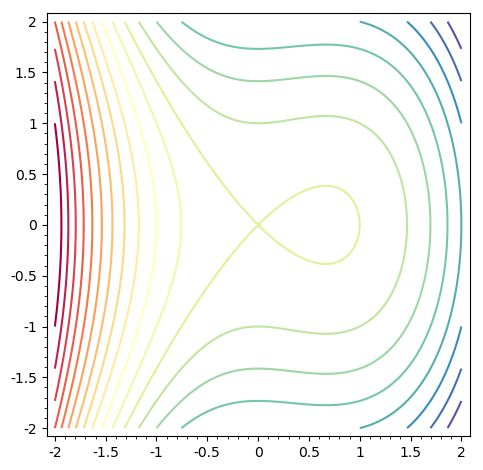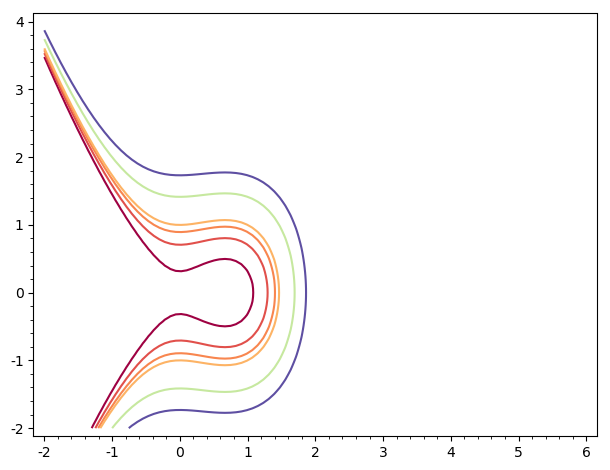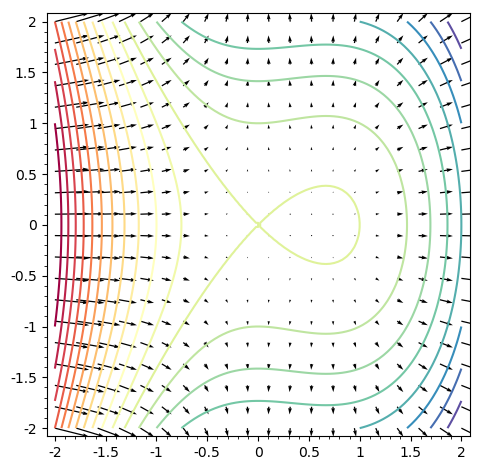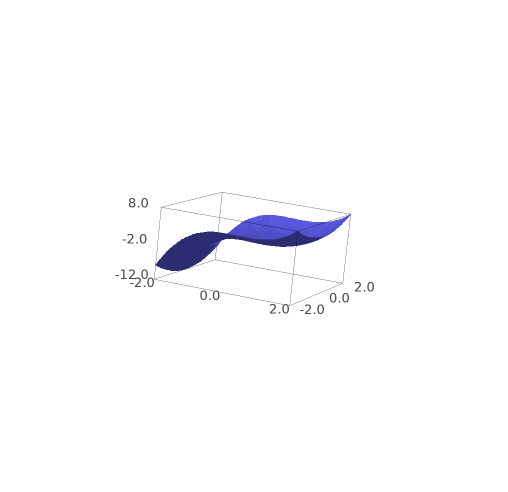# Revision history [back]

Since you didn't show the first and the second attempt to get g1, we can only guess.

var("x, y")
f(x,y)=x^3-x^2+y^2
# BTW, the correct name is "Spectral", not "spectral"...
g1 = contour_plot(f(x,y),(x,-2,2),(y,-2,2),
contours=20,fill=False,cmap='Spectral')
g1prime = contour_plot(f(x,y),(x,-2,6),(y,-2,4),
contours=[1/10,1/2,4/5,1,2,3],cmap='Spectral',fill=False)


for me, g1 isand g1prime isThey are obviously different, since you changed:

• the ranges, and
• the contours specification.

g2=plot_vector_field(f.gradient(), (x, -2, 2), (y, -2, 2))


to the first graph (same range specification) gives mewhich does not look that unreasonable...

Since you didn't show the first and the second attempt to get g1, we can only guess.

var("x, y")
f(x,y)=x^3-x^2+y^2
# BTW, the correct name is "Spectral", not "spectral"...
g1 = contour_plot(f(x,y),(x,-2,2),(y,-2,2),
contours=20,fill=False,cmap='Spectral')
g1prime = contour_plot(f(x,y),(x,-2,6),(y,-2,4),
contours=[1/10,1/2,4/5,1,2,3],cmap='Spectral',fill=False)


for me, g1 isand g1prime isThey are obviously different, since you changed:

• the ranges, and
• the contours specification.

g2=plot_vector_field(f.gradient(), (x, -2, 2), (y, -2, 2))